A Study on Orthogonal Space-time Block Codes for Cooperative Function | OMICS International
Journal of Electrical & Electronic Systems

# A Study on Orthogonal Space-time Block Codes for Cooperative Function

Sabran ON*

Department of Electronics and Communication Engineering, Bhabha College of Engineering, Bhopal, Madhya Pradesh, India

*Corresponding Author:
Sabran ON
Department of Electronics and Communication Engineering
Bhabha College of Engineering, Bhopal
Tel: +91-9203893960
E-mail: [email protected]

Received Date: August 06, 2015; Accepted Date: August 28, 2015; Published Date: September 15, 2015

Citation: Sabran ON (2015) A Study on Orthogonal Space-time Block Codes for Cooperative Function. J Electr Electron Syst 4:158. doi:10.4172/2332-0796.1000158

Copyright: © 2015 Sabran ON. This is an open-access article distributed under the terms of the Creative Commons Attribution License, which permits unrestricted use, distribution, and reproduction in any medium, provided the original author and source are credited.

Visit for more related articles at Journal of Electrical & Electronic Systems

#### Abstract

Space-time block codes alone generally have little or no coding gain. To extract coding gain, space-time block codes have been previously concatenated with an outer trellis to generate simple and powerful codes, recognized as superorthogonal codes. That work has two main themes: it explores methods and algorithms that generate coding gain in block codes exclusive of a trellis, as glowing as improve the coding gain in the presence of a trellis. At what time an external trellis is obtainable, our results simplify the super-orthogonal codes by finding new code supersets and corresponding set partitioning, resulting in improved coding gain. New algorithms are developed to efficiently build trellises for various full-rate MIMO codes, for that reason they extend the concept of trellis-block MIMO coding beyond orthogonal and quasi-orthogonal codes. In the absence of a trellis, a technique called single-block coded modulation is proposed to improve the coding gain of all varieties of space-time block codes. In that thesis they discuss coded modulation schemes designed for multiple antenna wireless channels without information of the channel at the transmitter. Space-time coding reduces the detrimental effect of channel fading. The space-time receiver takes advantage of diverse propagation paths between transmits and receive antennas to improve the performance of wireless communication. It contains a literature survey of the recent developments in MIMO signaling. The main types of spacetime codes are block and trellis codes. Space-time block codes (STBC) operate on a block of input symbols, producing a matrix output. Space-time block codes do not generally provide coding gain. Their main feature is the provision of diversity with a very simple decoding scheme. Concatenation of orthogonal space-time block codes (OSTBC) with an outer trellis has led to simple and powerful codes, known as super-orthogonal codes or STB-TCM.

#### Keywords

Cooperative communication; Two-way transmission; Cyclotomic Orthogonal Space-time Block Code (COSTBC)

#### Introduction

OSTBC have full diversity (nT*nR ) , but have little or no coding gain. To provide both diversity and coding gain that can specify a spacetime code that has an in-built channel coding mechanism then example space-time trellis codes, or one can choose a space-time block code concatenated with an outer channel code. Borran et al.  discussed design issues of concatenating channel codes with OSTBC. They show that design issues in maximizing diversity gain, and maximizing coding gain can be decoupled. Appropriate to that effortlessness, that structure has been accepted, e.g. in WCDMA standard. Design of concatenated trellis coded inflection (TCM) and OSTBC, and also show that that scheme outperforms space-time trellis codes with the same spectral efficiency, trellis complexity and signal constellation. That gives a new view of OSTBC over fading channel as an equivalent SISO channel. By means of that corresponding channel model, they give analytical evaluation of error probability, without considering the effect of block fading (which is typically assumed for linear decoding of STBC). To the give error bounds for MTCM-STBC under Rician Fading. However, interleaving does not appear in their analysis. Union bounds for channel codes and Alamouti signaling for temporally correlated and i.i.d channel. But again, the block fading assumption is absent in his analysis. None of the above mentioned works discuss spatially correlated fading. Simulate concatenated convolutional/turbo codes with two temporally and spatially correlated antennas in the structure of WCDMA, but do not present any investigation.

Introduction to Space-time coding

A typical communication system consists of a receiver, a transmitter and a channel. Space-time coding involves utilize of multiple transmit and receive antennas, as illustrated in Figure 1. Bits entering the space-time encoder serially are distributed to equivalent sub-streams. Surrounded by each sub-stream, bits are mapped to signal waveforms, which are then emitted starting the antenna corresponding to that substream. The system worn to map bits to signals is the called a space-time code. Signals transmitted simultaneously over each antenna interfere with each other as they propagate through the wireless channel. For now, the fading channel also distorts the signal waveforms. At the receiver, the distorted and superimposed waveforms detected by each receive antenna are used to estimate the original data bits.

That section deals with important characteristics of wireless channel and their mathematical models. They will first discuss about the flat fading channel and then they will go for the frequency selective fading channel which is more practical in broadband systems. Three assumptions they are considered like wide sense stationary, uncorrelated scattering and analogous correlation between two transmitting and receiving antenna depends only on the relative position of the antennas in spatial domain. Here a complex baseband signal is represented by its complex baseband representation. Figure 2 exhibits a complex baseband communication system.

MIMO overview

A wireless communication system with multiple transmitting and receiving antenna elements is called a MIMO organization. The principle of that setup is that transmit signals can be thus designed, and obtain signals so processed, that bit-error rate (quality) or data rate (bit/sec) of the communication is enhanced. MIMO signaling operates by spreading the information across both space and time. Signal processing in time is the natural dimension of the digital communication data. Spatial processing is possible through the use of multiple spatially distributed antennas. MIMO spatial processing takes advantage of multipath propagation, which is a key feature of wireless channel. Multipath fading has been habitually obscurity in wireless transmission. However, MIMO successfully takes advantage of random fading [2-6] and when available, multipath delay spread [7,8] for improving the quality of wireless communication. That improved performance requires no extra spectrum, but demands added hardware and complexity (Figure 3).

Multiple antennas: Multiple antennas are used at both the transmitter and receiver to improve the system performance in Rayleigh fading channel. That chapter deals with the technique of designing multiple antenna systems to exploring spatial diversity in order to improve the system performance by increasing the signal to noise ratio. Spatial diversity primarily focuses on the modification techniques in the receive side, with the aim of overcoming fading caused by multipath propagation (Figure 4).

From the above upper bound, they can define the diversity gain of the code as the ex- potent of SNR, i.e. rM, and coding gain as the multiplication of nonzero Eigen values. Therefore a good design criterion to guarantee full diversity is to make sure that for all possible code words C and E the matrix A(C,E) is full rank . Then to increase the coding gain for a full diversity code, an additional good design criterion is to maximize the minimum determinant of matrices A(C,E) for all C 6= E. The above two criteria for designing space-time codes are called rank and determinant criterion respectively (Figure 5).

#### Algorithm of Space Time

The overall capacity is the sum of capacities of each of these modes. Clearly, linear growth in the number of antennas is dependent on the properties of the eigen values. If they decay rapidly, then linear growth would not occur in practice. However, the eigen values have a known limiting distribution  that is unlikely that most eigen values are very small and the linear growth is indeed achieved. The capacity (2.1) is a random variable and does not give a single-number representation of channel quality. Two straightforward summaries are commonly used: the mean (or ergodic) capacity [9-11] and capacity outage [3,7,9,12]. Capacity outage measures (usually based on simulation) are often denoted C0:1 or C0:01, i.e., Now they can focus on the information theoretic capacity of a MIMO system. The MIMO signal model is

r = Hs + n

Where r is the Lr ×1 received signal vector, s is the Lt ×1 transmitted signal vector and n is an Lt ×1 vector of additive noise terms, assumed i.i.d. complex Gaussian with each element having a variance equal to σ 2 . For expediency they normalize the noise potheyr so in that chapter they assume σ 2 =1. Note that the system equation represents a single MIMO user communicating over a fading channel with additive white Gaussian noise (AWGN). The simply interference near is self-interference between the input streams to the MIMO system. Some authors have considered more general systems but most information theoretic results can be discussed in that simple context, so they use following equation as the basic system equation.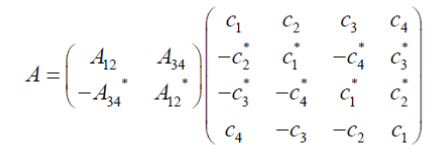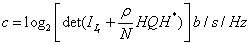For every input symbol sl, a space-time encoder generates Lt code symbols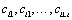. These Lt code symbols are transmitted simultaneously from the Lt transmit antennas. They define the code vector as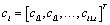Suppose that the code vector sequence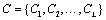was transmitted. They consider the probability that the decoder decides erroneously in favor of the legitimate code vector sequence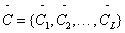Consider a frame or block of data of length L and define the Lt _ LtError matrix A as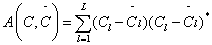If ideal channel state information (CSI)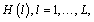is available at the receiver, then it is possible to show that the probability of transmitting C and deciding in favor of ~C is upper bounded for a Rayleigh fading channel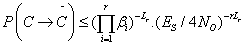Orthogonal space-time codes

The Alamouti space-time code supports maximum-likelihood (ML) detection with linear processing at the receiver. The simple structure and linear detection of that code makes it very attractive; it has been adopted for both the W-CDMA and CDMA- 2000 standards. That scheme was later generalized in  to an arbitrary number of antennas. Here, they will brief review the basics of STBCs. Figure 6 shows the baseband representation for Alamouti STBC with two antennas at the transmitter. The input symbols to the space-time block encoder are divided into groups of two symbols each. At a given symbol period, the two symbols in each group {c1,c2} are transmitted simultaneously from the two antennas. The signal transmitted from Antenna 1 is c1 and the signal transmitted from Antenna 2 is c2. In the next symbol period, the signal -c*2 is transmitted from Antenna 1 and the signal c*1 is transmitted from Antenna 2. They assume a single-antenna receiver, and denote with h1 and h2 be the channels from the and second transmit antennas to the receive antenna, (Figure 7) respectively. The channel gains are constant over two consecutive symbol periods. The received signals can be expressed as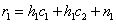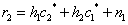where r1 and r2 are the received signals over two consecutive symbol periods and n1 and n2 represent the receiver noise and are modeled as complex Gaussian random variables with zero mean and potheyr spectral density No=2 per dimension. They define the received signal vector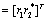the code symbol vector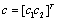, and the noise vector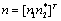. Equations (2.7) and (2.7) can be rewritten in a matrix form as

r = Hc + n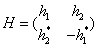The matrix H represents a concatenation of the channel vector (h1 h 2) t and the Alamouti code. The vector n is a complex Gaussian random vector with zero mean and covariance No = 2. Let us define C as the set of all possible symbol pairs c = {c1,c2 }. Diversity order provided by that scheme is 2Lr. Figure 7 shows a simplified block diagram for the receiver with two receive antennas. Note that the decision rule in (2.12) and (2.14) amounts to performing a hard decision on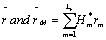respectively. Therefore, as shown in Figure 7, the received vector after linear combining, rM , can be considered as a soft decision for c1 and c2, which can be utilized by any outer channel codes used in the system. Note also that for the above 2×2 STBC, the transmission rate is one symbol/transmission, and it achieves the maximum diversity order of 4 that is possible with a 2×2 system. The method of Alamouti can be generalized to more than two transmit antennas [15-18]. The resulting orthogonal codes are still optimally decoded with a linear receiver. Unfortunately, only a few codes with a rate of one symbol/ transmission are available, and for the case of general complex-valued signals, there is no orthogonal rate-1 code beyond the Alamouti code . However, it is possible to design orthogonal codes by relaxing the rate requirement below one symbol/ transmission. For example, for Lt=4, a rate 1/2 STBC is given by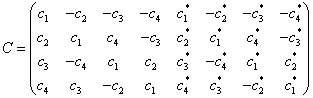Quasi orthogonal space-time codes

Earlier they saw that orthogonal codes allow a linear receiver, but in general they support a rate smaller than one symbol per transmission for Lt>2. Quasi-orthogonal codes compromise on a fully orthogonal code in order to achieve the full rate of one symbol per transmission for Lt>2 Recall that the Alamouti code is dinned by the following transmission matrix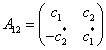Where the subscript  is to represent the in determinates c1 and c2 in the transmission matrix. Now, let us consider the following spacetime block code for four transmit antennas as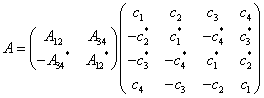#### Literature Review

Yudong et al.  investigated on a novel two-way cyclotomic orthogonal space-time transmission scheme (TCOSTS) is designed for asynchronous cooperative systems. In TCOSTS, the two terminals transmit signals to each other simultaneously to double the transmission rate. By exploiting cyclotomic orthogonal space-time block code (COSTBC), this scheme achieves full rate, full diversity and low decoding complexity. Also, higher diversity order is available by employing more relay nodes. Benefiting from OFDM asynchronous system architecture, operations implemented at relay nodes are very simple, and the scheme is tolerant of delays between relay nodes.

Qaja Walid et al.  studied a amplify-and-forward (AF) type cooperative wireless relay networks employing single bit closed-loop extended orthogonal space-time block coding (CL EO-STBC) over two selected cooperating relay nodes. Selection is performed from a set of NR available relay nodes each equipped with two antennas and outer convoluted coding is used to improve performance. A near-optimum detection scheme is used at the destination node for overcoming the effects of imperfect synchronization among selected relay nodes. End to-end simulation results show that the employed detection scheme can effectively eliminate the interference components induced by a synchronism with low detection complexity. Furthermore, the one-bit feedback scheme and relay selection technique can enhance the overall system performance and outperform previous feedback methods.

Chambers et al.  investigated on a modified quasi-orthogonal space-time block coding (M QO-STBC) scheme with full diversity and code gain distance (CGD) for use in asynchronous relay networks. They implement an orthogonal frequency division multiplexing (OFDM) scheme with cyclic prefix (CP) at the source to mitigate the effects of random delays between the relay nodes and the destination caused by a-synchronism. The modified code is structured by using a proper signal rotation and set partitioning of two quasi-orthogonal codebooks. Furthermore, the best two relays are selected from a set of M available relays based on the end-to-end instantaneous path gains. Simulation results show that the OFDM-based scheme can significantly enhance the performance of the system under imperfect synchronization. Simulations also show that the relay selection improved the reliability of the link as compared to a conventional system.

Shan Ding and Rui Li  studied a novel coding system which can reduce the error rate and improve the image transmission performance in the asynchronous cooperative MIMO systems. They propose a combined coder of LDPC-STBC with guard intervals for Set Partitioning in Hierarchical Trees (SPIHT) coded image transmission. The linear dispersion structure is employed to accommodate the cooperative wireless communication network in the dynamic topology of structure, as they will as achieving higher throughput than conventional space time codes based on orthogonal designs. The LDPC encoder without girth-4 and the STBC encoder with guard intervals are introduced respectively. The experiment results show that the combined scheme can be the good error correcting codes and achieve better BER performance in the asynchronous cooperative communication. The combined scheme can improve the quality of the reconstructed image in the PSNR values and SPIHT coded image transmission in the asynchronous cooperative MIMO systems.

Elazreg et al.  investigated on distributed space time block codes (D-STBCs) are applied within an asynchronous two-way cooperative wireless relay network using two relay nodes. A parallel interference cancelation (PIC) detection scheme with low structural and computational complexity is applied at the terminal nodes in order to overcome the effect of imperfect synchronization among the cooperative relay nodes. Simulation results based on end-to-end bit error rate (BER) illustrate that the PIC detection algorithm can mitigate the inter symbol interference (ISI) introduced by the a-synchronism, and that only a small number of iterations is necessary within the PIC detection to improve the system performance.

Mannai et al.  studied a new robust closed-loop extended orthogonal space-time block coding (EO-STBC) scheme for twoway four relay networks over frequency selective fading channels with imperfect synchronization. It is a three time slot scheme where the first one is specified for the two terminals while the other two are specified for amplify-and-forward (AF) relays. An orthogonal frequency division multiplexing (OFDM) data structure is employed at the two terminals using cyclic prefix (CP) insertion to combat the effect of multi-paths and time a-synchronicity. Full spatial diversity with array gain and code rate of 2/3 is achieved through applying a simple feedback approach over only two relays. Simulation results are used to show the performance improvements resulting from the proposed system. Furthermore, our scheme uses a simple symbol-wise maximum likelihood (ML) decoder to extract the information data.

#### Problem Description

The first challenge In general, diversity means using different dimensions of the channel, e.g. space, time, frequency, and so on, to get better the equivalent channel seen by the receiver. In that thesis they will specifically deal with one of the realizations of space diversity techniques termed as space-time codes. It is in general any modulation scheme which is designed for a multiple transmitter wireless system that tries to achieve antenna (space) range.

The problem of designs of space-time codes they are in the form of trellis coded modulation, and beginning exponential decoding complexity as the number of transmit antennas increased. After a while, simple transmitter diversity scheme which benefitted both full diversity of a two-transmit antenna channel as they will as simple Maximum- Likelihood (ML) decoding. The superior properties of that code inspired to inspect the existence of similar designs for more numbers of transmit antennas. In the container of complex codes, i.e. modulation schemes using complex constellation members, the authors proposed a structured modulation method, called Orthogonal Space-time Block Code that could send on average one symbol in every two time slots, in addition to achieved full diversity as they will as simple ML decoding.

Motivation

Although our intent is to present an analysis that is general and comprehensive. They will broaden existing results. They are supposing that the channel conditions are known exactly to the receiver, i.e. they have perfect channel state-information (CSI). They present the analysis in two parts. In investigate concatenated channel codes and orthogonal space-time block codes. That provides full diversity as they will as coding grow. As the outer codes, they consider convolutional codes, turbo codes, TCM and MTCM. Inside the succeeding part, they at hand generalized analysis for all space-time codes.

#### Conclusions

They recommend a new space-time code design technique by pruning code words from an expanded set of orthogonal space-time code words. They select the code words from the expanded set through an expurgation algorithm. The consequential codes exhibit attractive coding gains, while maintaining very reasonable decoding density. It is enlightening to revisit the varieties of space-time codes designed for diversity that also provide coding gain. The rest type is trellis spacetime codes, which allow coding gain but whose complexity is generally higher than block space-time codes.

The super-orthogonal codes of which are constructed by expanding block codebooks (e.g Alamouti) and building trellises on them. That is third type consists of codes presented in that function, which incorporate coding gain into the block code itself. Because they do not involve a trellis, this decoding complexity is smaller than either.

Than exploring a new family of codes known as Space-time codes for transmission in wireless Rayleigh fading channel using multiple antennas at either the transmitter or receiver side. Many subfamilies of space–time codes they are also set up. They examine the performance of these codes and a comparison between them is completed. Space– time codes can be readily implemented in DSP due to their simplicity in architecture and design. In addition to the performance of these codes they have also studied the diversity feature of each code.

#### Future Work

The Space Time Coding could be implemented in distributed networks along with the Randomization system where each node of the network transmit an autonomous, arbitrary linear combination of the columns of a space time code matrix, irrespective of the number of cooperative node. That approach enables a novel design of the line to decentralize the relay transmission and yet obtain the same diversity as that of multi antenna organization. A distributed planning is advantageous in that there is no single point of failure, with the potential for continuous operation in the presence of individual terminal or node failures. They can also apply the STC to frequency selective channel.

#### References

Select your language of interest to view the total content in your interested language

### Article Usage

• Total views: 11008
• [From(publication date):
December-2015 - Jan 21, 2020]
• Breakdown by view type
• HTML page views : 10757Can't read the image? click here to refresh